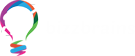### Vedic Maths#### Overview

Contains all shortcut methods of math. It is a collection of techniques to solve mathematical arithmetics in an easy way.

• Using regular mathematical steps, solving problems sometimes are complex and time-consuming. But using Vedic Mathematic’s General Techniques (applicable to all sets of given data) and Specific Techniques (applicable to specific sets of given data), numerical calculations can be done very fast.
• It can eradicate the fear of Math completely. So If your child has Math-Phobia High-Speed Vedic Maths is a Fun-Filled way to do Math and arises interest in your child.

• Stars 5
• %0
• Stars 4
• %0
• Stars 3
• %0
• Stars 2
• %0
• Stars 1
• %0

## 0.0

Course Rating

#### Reviews

You will get 50% Off on the Next Order
2 / 1 Year

•   Engaging educational opportunities
•   Self improvement & Personal growth

##### Includes

This main category includes

• High-Speed Multiplication
• Faster Division
• Algebra: Linear, Simultaneous, Quadratic and Miscellaneous Equations
• Instant Subtractions
• Squares
• Cubes
• Square Roots
• Cube Roots
• Digital Roots
• Math Meditation - Doing Math Calculations without Pens and Paper!
• Divisibility
• Calendars
• Pythagoras Theorem
• Recurring Decimals
• Determinants
• Triples
• Coordinate geometry
• Calculus